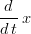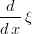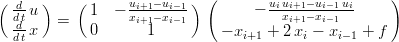## Tuesday, June 16, 2009

### Burgers' Equation on a Moving Grid

The familiar inviscid Burgers' equation on a non-moving, physical-space coordinate system:Now we want to transform the equation, which is easily done in Maxima:

depends([u, x], [t]);
depends([xi], [x]);
depends([u], [xi]);

burgers : 'diff(u, t) + u * 'diff(u, x);

ev(burgers, nouns);

This gives us Burgers' equation transformed to computational space and a moving grid:Now we've got some extra terms. The grid velocity:And the grid transformation (to go from the computational space to the physical space):The grid velocity is the troublesome term, because while we have an equation governing the time evolution of the unknown, we don't have a governing equation for the grid. This is where moving grids get fun, we get to pick the governing equation. How about a forced, unsteady, linear diffusion equation?The method of lines is a popular and practical way to formulate PDEs for numerical solution. In Maxima, it is very straightforward to substitute in some finite difference expressions for the spatial derivatives, to get our semi-discrete equation for the time-derivatives.

/* substitute central differences for the spatial derivatives: */
burgers_discrete_space : ratsubst(u[i+1] - u[i-1], 'diff(u, xi), burgers_trans);
burgers_discrete_space : ratsubst(1/(x[i+1] - x[i-1]), 'diff(xi, x), burgers_discrete_space);

/* governing equation for the grid (forced diffusion equation): */
grid : 'diff(x,t) + 'diff(x, xi, 2) = f;
/* substitute central differences for the spatial derivative: */
grid_discrete_space : ratsubst(x[i+1] - 2*x[i] + x[i-1], 'diff(x,xi,2), grid);

The reason for transforming to computational coordinates is because the nodes are (usually) equally spaced in the computational domain. That way we can use the same difference formula for every point no matter what the spacing is in the physical space.

Now we can use the augcoefmatrix() function to get an operator and right-hand-side vector for our rate equation.

Rate_Operator : augcoefmatrix([burgers_discrete_space, grid_discrete_space], ['diff(u,t), 'diff(x,t)]);
Rate_RHS : -submatrix(Rate_Operator, 1, 2);
Rate_RHS : ratsubst(u[i], u, Rate_RHS);
Rate_Operator : submatrix(Rate_Operator, 3);
Rate_Operator_inv : invert(Rate_Operator);
Rate_Vector : transpose(['diff(u,t), 'diff(x,t)]);
Rate_expression : fullratsimp(Rate_Operator_inv.Rate_RHS);

Here's the resulting semi-discrete equations:Because our choice of governing equation for the grid doesn't depend on the time-derivative of the solution, the operator is easily inverted:and then applied, to give a vector of nonlinear expressions for our rates:Note that in the absence of any forcing, the steady state grid is equally distributed grid points. The forcing function is what allows us to cluster grid points where higher resolution is needed. This forcing function should be based on some measure of the local error in the numerical solution. It is convenient just to use magnitude of the solution derivative since we've already calculated it, and areas with high gradients tend to need more points to resolve well.

Equal spacing in computational space is standard for using finite differences, but what about spectral methods where we usually choose nodes according to the Chebyshev polynomial roots? In this case it is convenient to choose our computational spacing to be the roots spacing rather than equal spacing. Here's the derivative of the coordinate with respect to computational coordinate using the DCT derivative routine.Which shows that the roots nodes give a constant grid transform while the equally spaced nodes have a non-constant transform (opposite the normal situation of equally spaced computational coordinates).